### 14.6.3.4 Computing optimal solutions via dynamic programming

Dynamic programming with interpolation, as covered in Section 14.5, can be applied to solve the problem once it is formulated in terms of the path-constrained phase space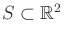. The domain of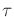provides the constraint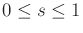. Assume that only forward progress along the path is needed; moving in the reverse direction should not be necessary. This implies that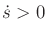. To make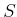bounded, an upper bound,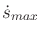, is usually assumed, beyond which it is known that the speed is too high to follow the path.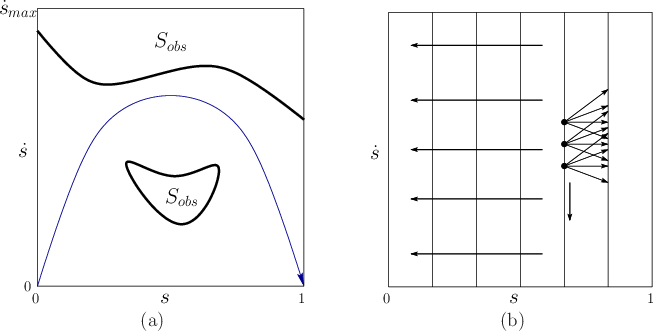This results in the planning problem shown in Figure 14.27a. The system is expressed as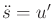, in which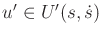. The initial phase inis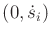and the goal phase is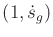. Typically,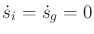. The region shown in Figure 14.27 is contained in the first quadrant of the phase space because only positive values of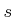and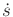are allowed (in Figure 14.13,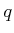and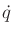could be positive or negative). This implies that all motions are to the right. The actions determine whether accelerations or decelerations will occur.

Backward value iteration with interpolation can be easily applied by discretizingand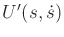. Due to the constraint, making a Dijkstra-like version of the algorithm is straightforward. A simple wavefront propagation can even be performed, starting at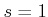and progressing backward in vertical waves until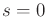is reached. See Figure 14.27b. The backprojection (14.29) can be greatly simplified. Suppose that the-axis is discretized into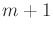regularly spaced values,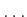,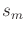at every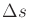, for some fixed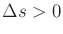. Thus,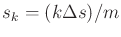. The indexcan be interpreted as the stage. Starting at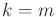, the final cost-to-go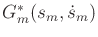is defined as 0 if the corresponding phase represents the goal, and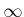otherwise. At each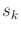, thevalues are sampled, and the cost-to-go function is represented using one-dimensional linear interpolation along the vertical axis. At each stage, the dynamic programming computation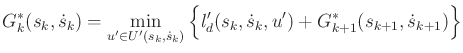(14.46)

is performed at eachsample. This represents a special form of (14.27). Linear interpolation over discretizedvalues is used to evaluate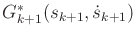. The cost term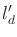is obtained from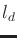by computing the original state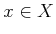fromand; however, if the trajectory segment enters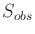, it receives infinite cost. The computations proceed until stage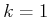, at which time the optimal cost-to-go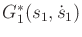is computed. The optimal trajectory is obtained by using the cost-to-go function at each stage as a navigation function.

The dynamic programming approach is so general that it can even be extended to path-constrained trajectory planning in the presence of higher order constraints . For example, if a system is specified as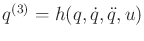, then a 3D path-constrained phase space results, in which each element is expressed as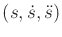. The actions in this space are jerks, yielding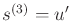for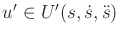.

Steven M LaValle 2020-08-14